B样条的动机来源于插值中的Runge-Kutta现象，高阶多项式很容易产生不稳定的上下波动。而样条思想，就是用分段低阶多项式通过连续的连接来代替高阶的多项式。

## B样条曲线及其性质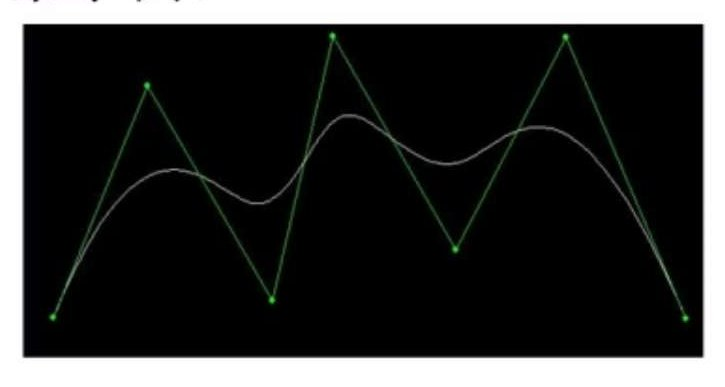B样条基函数定义有多种解释，最简单的是由de Boor-Cox递推公式定义的：

• B样条基函数$N_{i,k}(t)$的非零区间是什么？
从上述可以看到，当$k=0$时，$N_{i,0}(t)$的非零区间为：$(t_i - t_{i+1})$。根据递推公式，可以得到：

• 一共需要多少个节点？

$N_{i,k}(t)$的非零区间是$(t_{i},t_{i+k+1})$，共有$n$个控制节点，因此所有的非零区间为：$(t_{0},t_{k+1})\cup (t_{1},t_{k+2})\cup…\cup (t_{n},t_{n+k+1})$。因此需要的节点个数为$n+k+2$。

• B样条插值得到的曲线定义区间是什么？
这个也很容易得到：$(0,t_{n+k+1})$。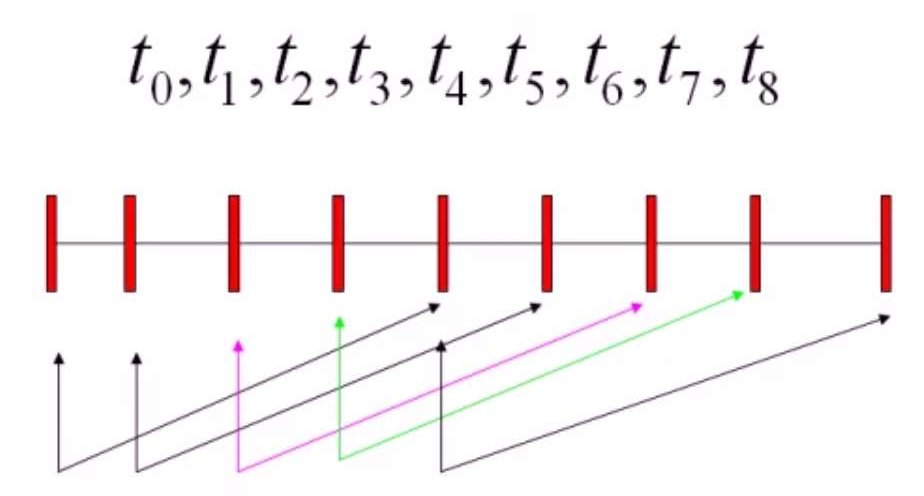### B样条基函数性质

B样条基函数有很多性质与Bezier曲线类似。

• 非负性和局部支撑性
$N_{i,k}(t)$是非负的，$N_{i,k}(t)$是$[t_i,t_{i+k+1}]$上的分段非零多项式：
• 归一性
区间$[t_i,t_{i+1}]$上的所有$k$次非零基函数的和为1：

• 基函数所满足的微分方程：

### B样条分类

• 均匀B样条。节点成等差数列均匀分布，这样的节点分布对应的是均匀B样条基函数。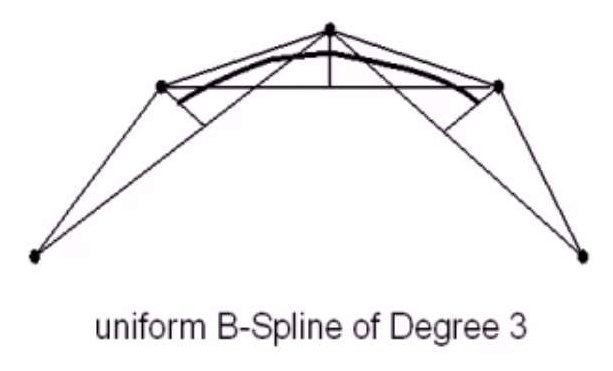• 准均匀B样条。准均匀B样条的起始节点和终止节点都具有$k+1$的重复度,其他的节点和均匀B样条一致。均匀B样条不保持Bezier曲线的断电性质，起始点和终止点不在控制端点上。而准均匀B样条具有端点性质。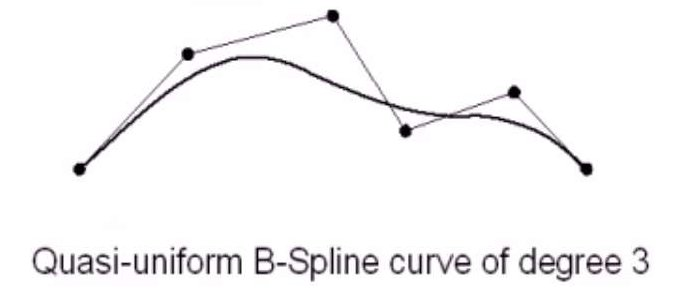• 分段Bezier曲线。
除了终点和起始节点具有k+1个重复度，其他的节点具有$k$个重复度，这样得到的实际上是分段的Bezier曲线。此时需要节点个数满足：$(n+k+2-2)%k=0$。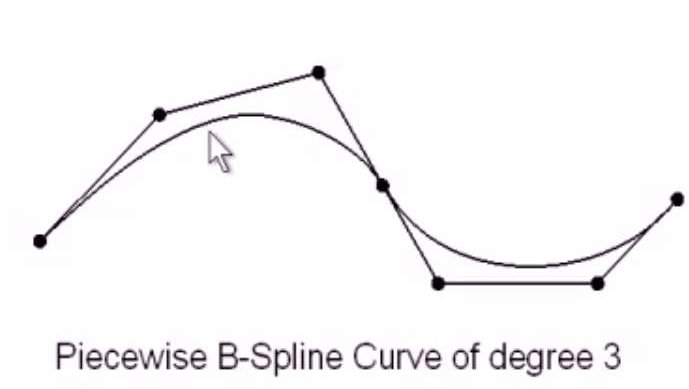• 非均匀B样条。最一般的B样条，我们主要介绍这个。它的起始节点和终止节点的重复度小于等于$k+1$，而其他节点重复度小于$k$。

### 非均匀B样条

B样条曲线的性质：

• 局部支持性。区间$t \in [t_i,t_{i+1}]$上的曲线仅由至多$k+1$个控制点决定。修改控制点$P_i$仅会影响到$(t_i,t_{i+k+1})$上的曲线。

• 连续性：$P(t)$在每一个重复度为$r$的节点上具有$C^{k-r}$的连续性。不知道在说什么。

• 凸包性。B样条曲线被包围在其控制顶点的凸包内部。

• 分段多项式性质：任何一个由相邻节点确定的区间上，$P(t)$是一个关于t的次数不超过$k$的多项式。

• 导数公式：
• 变差缩减性：任何一条直线与B样条曲线的交点个数不会超过该直线与B样条曲线的控制多边形的交点数目
• 几何不变性：曲线的形状和相对于控制点的位置不取决于坐标系的选择
• 仿射不变性：将仿射变换用于控制点，变换后的控制点得到的曲线就是仿射变换后的曲线。
• 直线保持性：如果控制多边形退化成一条直线，那么B样条曲线在这条直线上
• 灵活性：使用B样条可以方便构成线段，尖点，切线等等特殊效果。对于灵活性有下面几个3次B样条基函数的例子：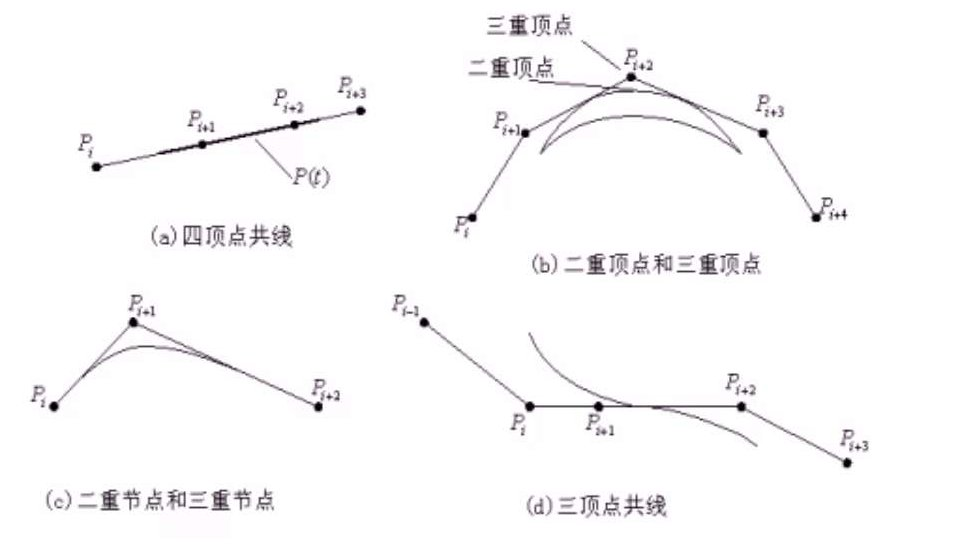### de Boor算法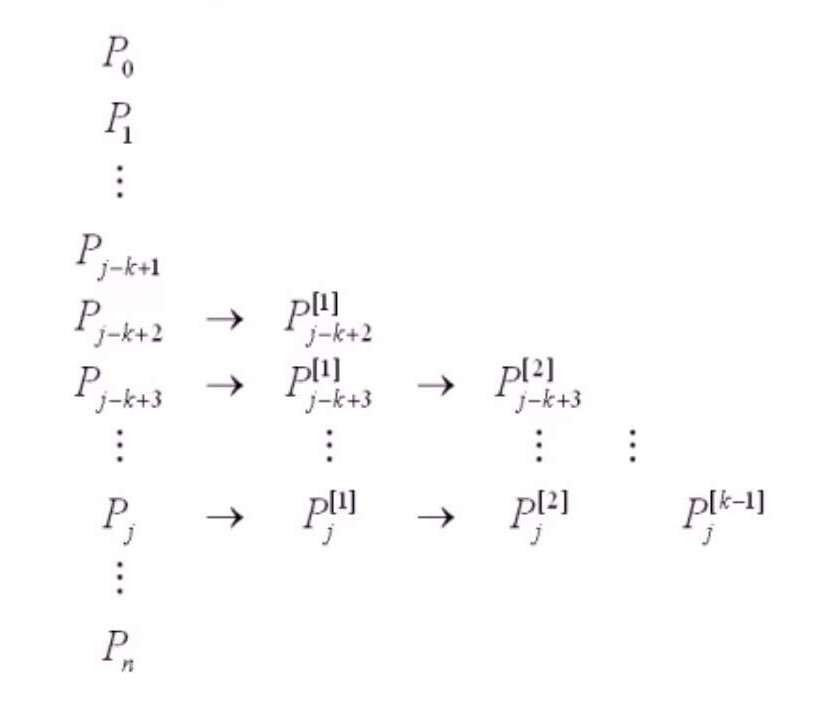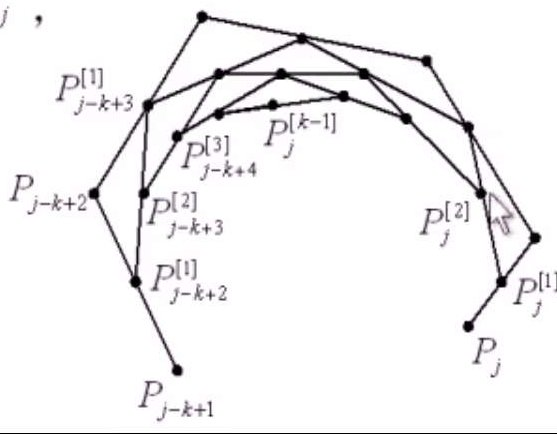### 节点插入

• 插入新的节点$t$到节点区间$[t_i,t_{i+1}]$
• 节点向量变为：$T^1 = [t_0,t_1,…,t_i,t,t_{i+1},…,t_{n+k+1}]$
• 新的节点向量变为：$T^1 = [t^1_0,…,t^1_i,t^1_{i+1},t^1_{i+2},…,t_{n+k+2}]$

$r$是新插入的节点$t$在节点序列中的重复度。如下图（图中k为阶数）：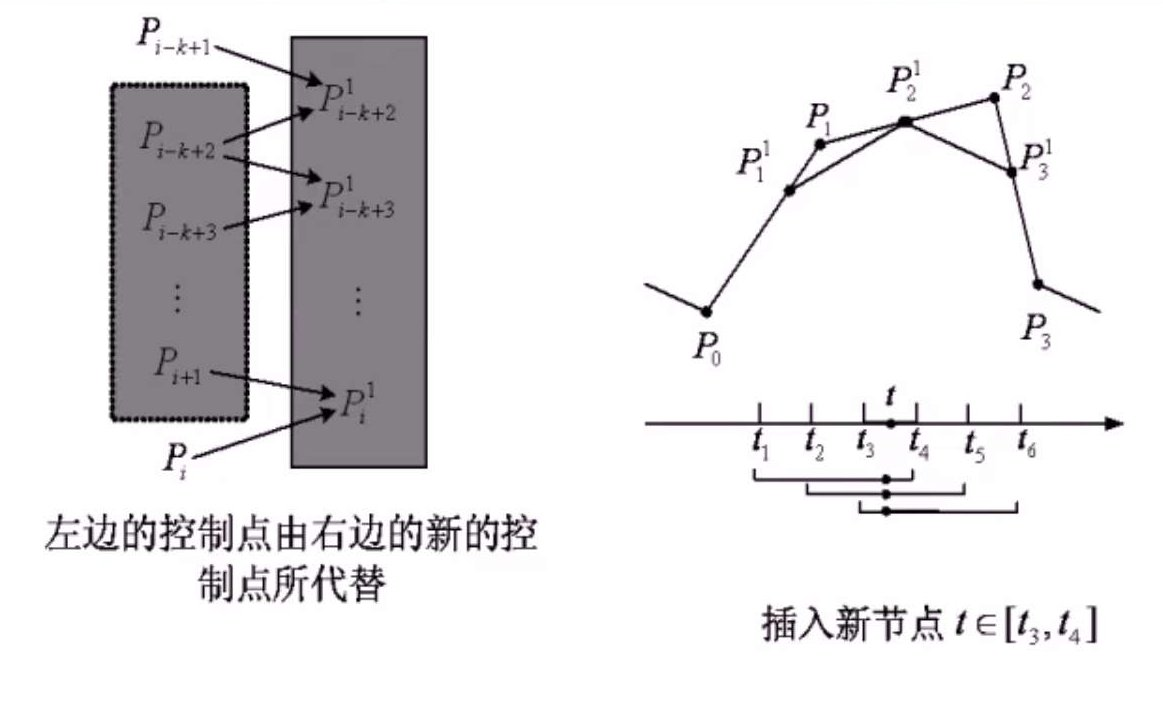## B样条曲面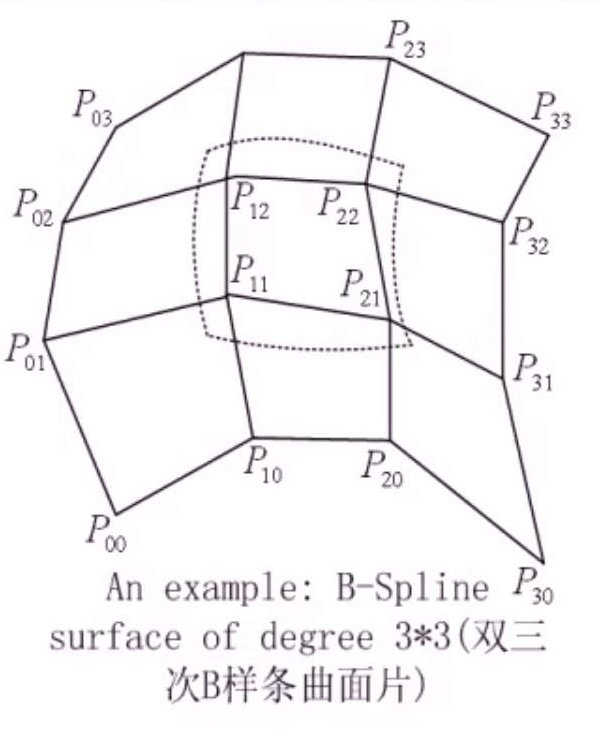## NURBS曲线/曲面

B样条和Bezier都有缺点，不能精确表示圆锥曲线。而NURBS（非均匀有理B样条）目的在于找到能精确描述圆锥曲线以及二次曲面的方法。在这里简单介绍一下NURBS。

NURBS有下面几个优势：

• 它提供了一个更一般更精确的方法来表达并对自由曲线/曲面进行设计。
• 提供了一个通用的数学形式，可以同时表示标准的解析曲线/曲面（如圆锥曲线）以及自由曲线/曲面（如参数曲线）。
• 存在稳定快速的数值计算方法。
• 具有仿射变换不变性，也有投影变换不变性
• 对于NURBS的控制点和权重都能任意修改，用NURBS来做设计有更大的灵活性
• 非有理B样条，非有理和有理Bezier曲线/曲面都可以看作NURBS的特殊形式
它的缺点是比传统的表达需要更大的存储空间，而且如果权重设计不合理，NURBS曲线可能会产生畸变。在某些情况下如曲线重叠，使用NURBS非常难以处理。

NURBS曲线是由分段有理B样条多项式基函数定义的：

#### 圆锥曲线的NURBS表示

• $C_{sf} = \frac{\omega_1^2}{\omega_0\omega_2}$是形状因子，决定了圆锥曲线的类型。当$C_{sf}=1$时，曲线为抛物线。而对于$C_{sf}<1$，曲线为椭圆，当$c_{sf}=0$，曲线退化为一条直线，当$c_{sf}>1$，曲线为双曲线，当$C_{sf} \rightarrow \infty$，曲线退化为两条直线。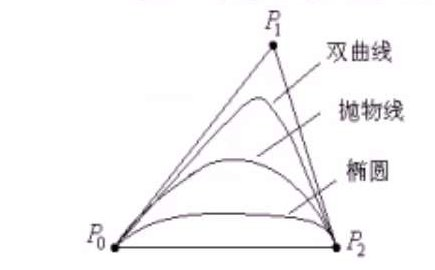#### NURBS的修改

NURBS曲面的定义也就是曲线简单的拓展：

NURBS曲面也具有可微性，同时存在局部极值点，也具有和B样条曲面类似的多种性质。这里就不过多介绍了。